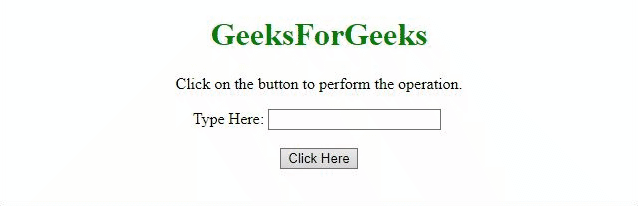Related Articles
How to convert first letter of a string to upper case using jQuery ?
• Last Updated : 27 Apr, 2020

The task is to capitalize the first letter of string without using toUpperCase() method with the help of jQuery. There are two approaches that are discussed below:

Approach 1: In this example, the css() method is used to used to set the text-transform property value to capitalize.

Example:

 ` ``<``html``> `` ` `<``head``> ``    ``<``title``> ``        ``How to convert first letter of a``        ``string to upper case using jQuery?``    ````     ` `    ``<``script` `src``=``"https://ajax.googleapis.com/ajax/libs/jquery/3.4.0/jquery.min.js"``>``    ```` `` ` `<``body` `style` `= ``"text-align:center;"``> ``     ` `    ``<``h1` `style` `= ``"color:green;"` `> ``        ``GeeksForGeeks ``    ````     ` `    ``<``p``>``        ``Click on the button to``        ``perform the operation.``    ````     ` `    ``Type Here: <``input` `id` `= ``"input"``/>``    ``<``br``><``br``>``     ` `    ``<``button` `onclick` `= ``"GFG_Fun()"``>``        ``Click Here``    ````     ` `    ``<``p` `id` `= ``"GFG"``>``     ` `    ``<``script``>``        ``var geeks = document.getElementById('GFG');``         ` `        ``function GFG_Fun() {``            ``\$('#input').css('textTransform', 'capitalize');``            ``geeks.innerHTML = "Text is capitalized";``        ``}``    `` `` `` ` ``

Output:Approach 2: In this example, we are using CSS property to perform the operation. A new ID has been added to the element which sets the property text-transform to capitalize.

Example:

 ` ``<``html``> `` ` `<``head``> ``    ``<``title``> ``        ``How to convert first letter of a``        ``string to upper case using jQuery?``    ````     ` `    ``<``script` `src``=``"https://ajax.googleapis.com/ajax/libs/jquery/3.4.0/jquery.min.js"``>``    ````     ` `    ``<``style``>``        ``#capital {``            ``text-transform: capitalize;``        ``}``    ```` `` ` `<``body` `style` `= ``"text-align:center;"``> ``     ` `    ``<``h1` `style` `= ``"color:green;"` `> ``        ``GeeksForGeeks ``    ````     ` `    ``<``p``>``        ``Click on the button to``        ``perform the operation.``    ````     ` `    ``Type Here: <``input` `id` `= ``"input"``/>``    ``<``br``><``br``>``     ` `    ``<``button` `onclick` `= ``"GFG_Fun()"``>``        ``Click Here``    ````     ` `    ``<``p` `id` `= ``"GFG"``>``     ` `    ``<``script``>``        ``var geeks = document.getElementById('GFG');``         ` `        ``function GFG_Fun() {``            ``\$('#input').attr('id', 'capital');``            ``geeks.innerHTML = "Text is capitalized";``        ``}``    `` `` `` ` ``

Output:Attention reader! Don’t stop learning now. Get hold of all the important DSA concepts with the DSA Self Paced Course at a student-friendly price and become industry ready.

My Personal Notes arrow_drop_up